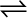7.23 At 1127 K and 1 atm pressure, a gaseous mixture of CO and CO2 in equilibrium with soild carbon has 90.55% CO by mass

C (s) + CO2 (g)2CO (g)

Calculate Kc for this reaction at the above temperature.

Let the total mass of the gaseous mixture be 100 g.
Mass of CO = 90.55 g
And, mass of= (100 – 90.55) = 9.45 g

Now, the number of moles of CO

Number of moles of

The partial pressure of CO,

The partial pressure of ${\mathrm{CO}}_{2}$,

Therefore ${K}_{p}$=

For the given reaction,